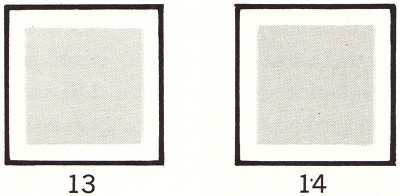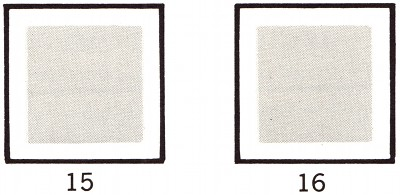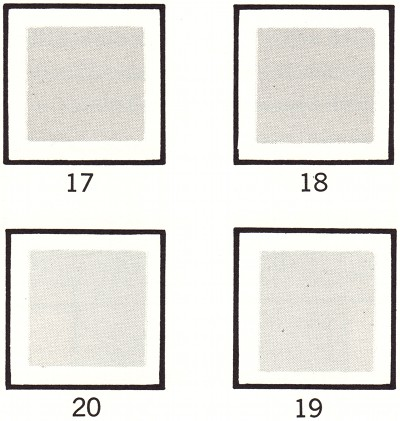## Ostwald on the Threshold

### September 21st, 2009

Three illustrations by Wilhelm Ostwald from his 1916 The Color Primer (Die Farbenfibel).

Continuity. Between two different grays it is always possible to insert a third gray, which is lighter than one and darker than the other. In this manner the steps can be made even smaller, until they finally become imperceptible.

“It probably follows that the complete gray series consists of an infinite number of steps. However, if one places between two terminal points a series of gray sheets, each of which is just noticeably lighter than the previous one, it will be found that one cannot discern an infinite number of intermediate steps. Rather, a finite difference is necessary if one is to distinguish a series of grays, and if the steps become very small, differences can no longer be discerned.

The Threshold. This border between just noticeable difference in color is called the threshold” (p20-21).“Thus, there is between 13 and 14 a just noticeable difference, while between 15 and 16 there is an unnoticeable difference. Even though 16 is a trifle weaker than 15, they both appear equally as light” (p21).Equality. Only the presence of the threshold makes it possible for us to regard two gray colors as equal. What we cannot distinguish we call equal [my emphasis]. Even if we could recognize every difference that actually existed (in gradations) it would be impossible to create two equal grays, as we could never remove the last traces of the actually existing differences. In effect, we will regard two gray colors such as 15 and 16 as equal, even though an objective difference between them has intentionally been created.

“The presence of the threshold has certain consequences with regard to the apparent equality of colors. These consequences are different from the mathematical relationships that are usually established when no regard is paid to the threshold. For example, in general this law applies: if a = b and b = c, then a = c. And it also follows that from a = b, b = c, c = d, that a = d. Now, if gray b is indeed lighter than a, but by less than the threshold, and if the same applies between c and b, and between d and c, we would first state a = b, b = c, and c = d. But if the sum of these imperceptible differences exceeds the limits of the normal threshold, then we could by no means say that a = d, but we would experience d as lighter than a” (p21-22).“Thus, there exists a difference between steps 17 and 18 that is smaller than the threshold. Therefore, if steps 19 and 20 are covered, 17 and 18 will appear equal. Similarly, 18 and 19 appear equal if 17 and 20 are covered, and the same applies to 19 and 20. We have observed, therefore: 17 = 18, 18 = 19, and 19 = 20 and are thus inclined to conclude also that 20 = 17. However, if we cover 18 and 19 and compare 20 and 17, step 20 is unmistakably lighter than 17.

“Incidentally, the threshold is not an invariable value, as it often has a different value with different persons. Much depends on visual ability; in some individuals the threshold may increase or shrink through exercise or through fatigue and other weakening influences. For this reason, some will not experience the difference between steps 13 and 14, while others will notice a difference between 15 and 16. The number of distinguishable steps of gray under normal conditions amounts to several hundred” (p23).

### One Response to “Ostwald on the Threshold”

1. Andrew Clarke Says:

The first thing that comes to mind is that this would make a great biology lesson. People have this assumption that nature respects our classification of it, and that every animal is equal to every other within the boundaries of specieshood. It makes it difficult for them to conceive of how a species could ever cross the gap and transition into another.

In actuality life is a gradient filled with colors that fall below the threshold. A can breed with B and B can breed with C and C can breed with D. But as the differences between them add up it’s often the case that D cannot breed with A. The contrast becomes perceptible and so we draw a line as best we can, but the transition is never made in a single step.